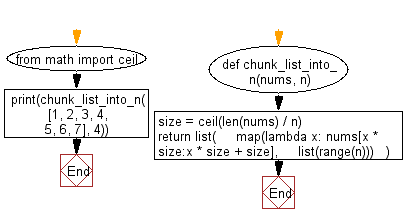﻿ Python: Chunk a given list into n smaller lists - w3resource# Python: Chunk a given list into n smaller lists

## Python List: Exercise - 233 with Solution

Write a Python program to chunk a given list into n smaller lists.

• Use math.ceil() and len() to get the size of each chunk.
• Use list() and range() to create a new list of size n.
• Use map() to map each element of the new list to a chunk the length of size.
• If the original list can't be split evenly, the final chunk will contain the remaining elements.

Sample Solution:

Python Code:

``````from math import ceil

def chunk_list_into_n(nums, n):
size = ceil(len(nums) / n)
return list(
map(lambda x: nums[x * size:x * size + size],
list(range(n)))
)
print(chunk_list_into_n([1, 2, 3, 4, 5, 6, 7], 4))
```
```

Sample Output:

```[[1, 2], [3, 4], [5, 6], ]
```

Flowchart:## Visualize Python code execution:

The following tool visualize what the computer is doing step-by-step as it executes the said program:

Python Code Editor:

Have another way to solve this solution? Contribute your code (and comments) through Disqus.

What is the difficulty level of this exercise?

Test your Python skills with w3resource's quiz

﻿

## Python: Tips of the Day

Floor Division:

When we speak of division we normally mean (/) float division operator, this will give a precise result in float format with decimals.

For a rounded integer result there is (//) floor division operator in Python. Floor division will only give integer results that are round numbers.

```print(1000 // 300)
print(1000 / 300)```

Output:

```3
3.3333333333333335```